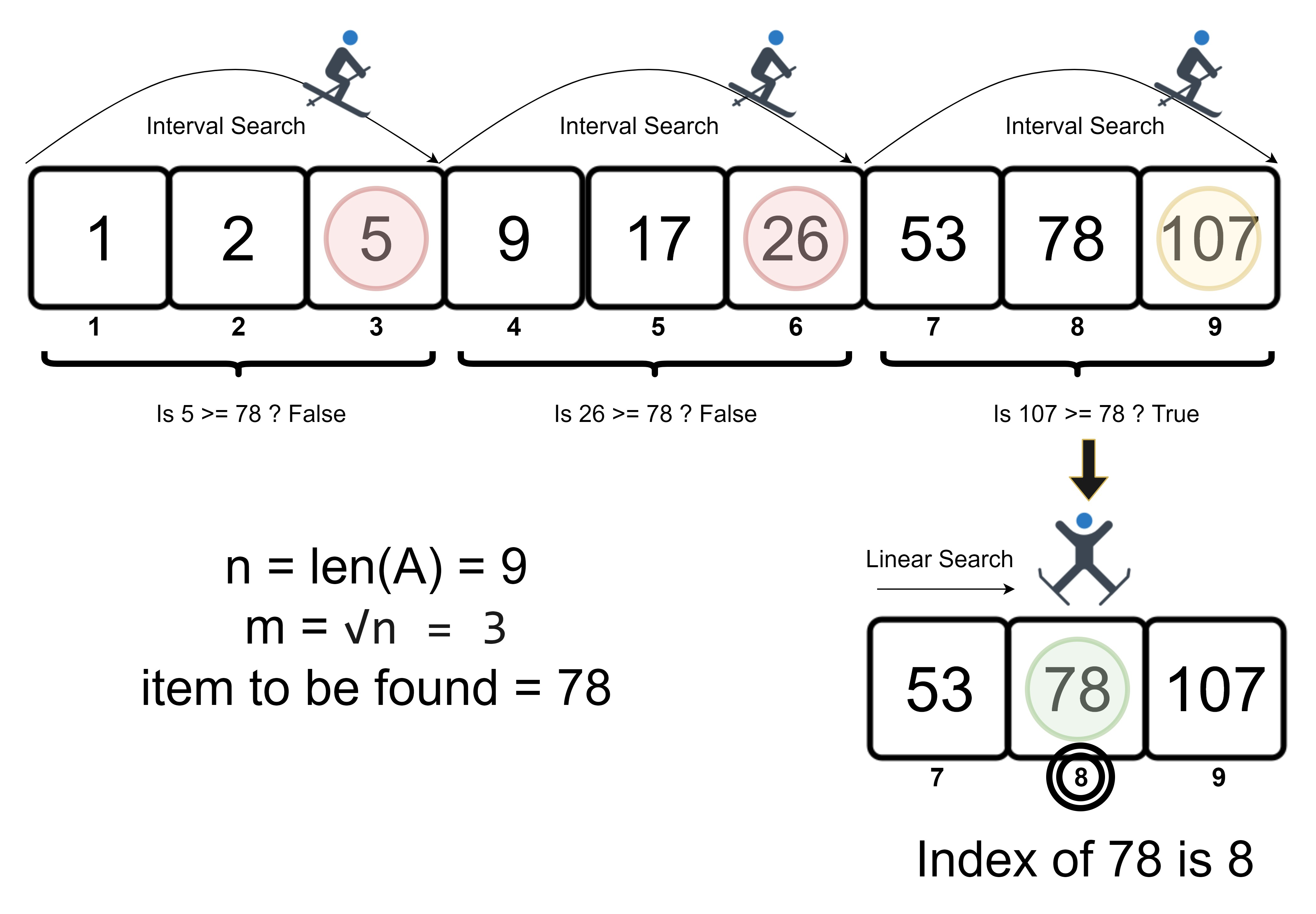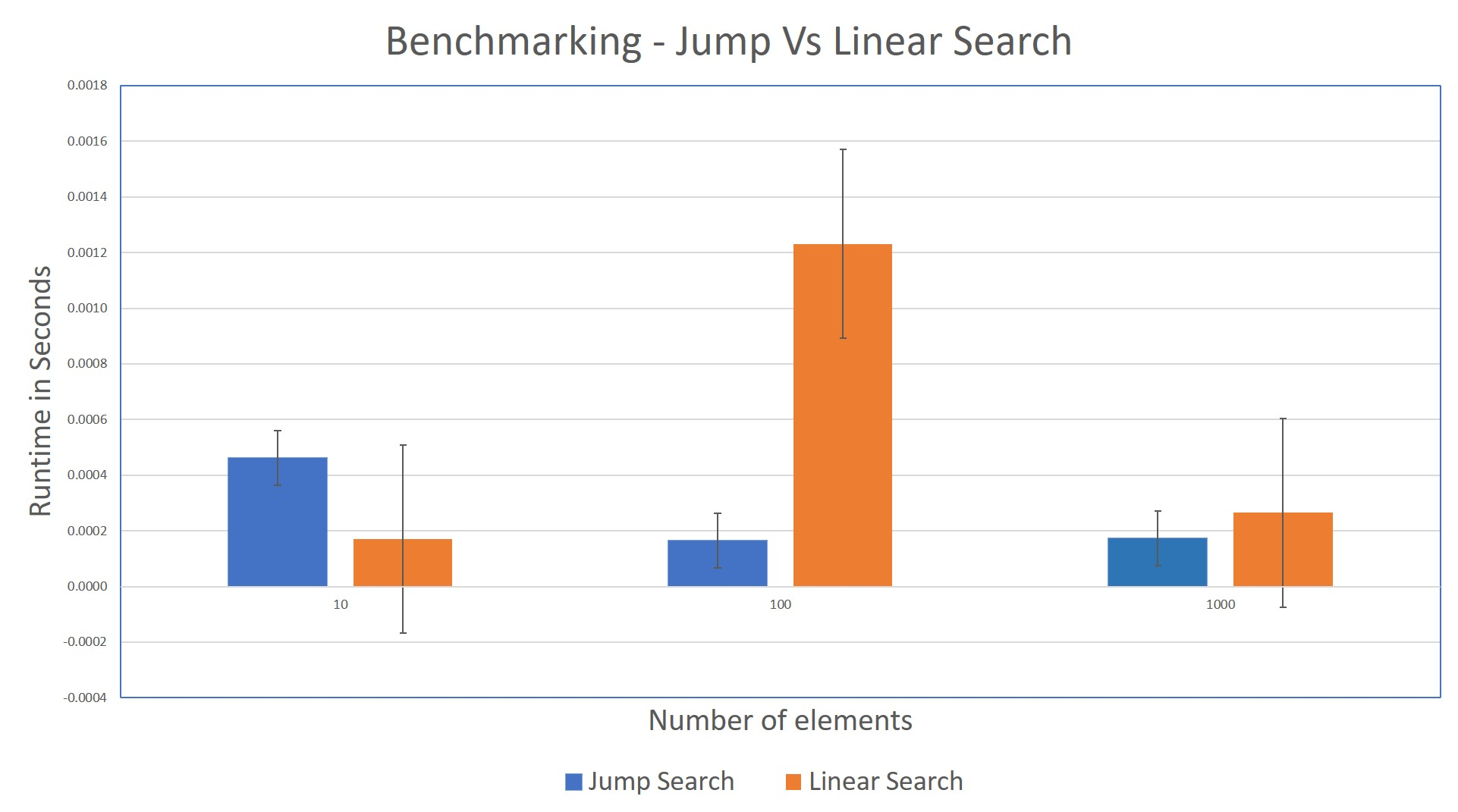Jump Search in Python - Stack Abuse

# Jump Search in Python### Introduction

Finding the right data we need is an age-old problem before computers. As developers, we create many search algorithms to retrieve data efficiently.

Search algorithms can be divided into two broad categories: sequential and interval searches. Sequential searches check each element in a data structure. Interval searches check various points of the data (called intervals), reducing the time it takes to find an item, given a sorted dataset.

In this article, you will cover Jump Search in Python - a hybrid combination of sequential search and interval search on sorted arrays.

### Jump Search

With Jump Search, the sorted array of data is split into subsets of elements called blocks. We find the search key (input value) by comparing the search candidate in each block. As the array is sorted, the search candidate is the highest value of a block.

When comparing the search key to a search candidate, the algorithm can then do 1 of 3 things:

1. If the search candidate is lesser than the search key, we'll check the subsequent block
2. If the search candidate is greater than the search key, we'll do a linear search on the current block
3. If the search candidate is the same as the search key, return the candidate

The size of the block is chosen as the square root of the array's length. Therefore, arrays with length n have a block size of √n, as this on average gives the best performance for most arrays.

It might be useful to illustrate how it works. Here's how Jump Search would fine the value 78 in an array of 9 elements:The above example finds the element in 5 steps, as there are two checks in the linear search section.

Now that we have a high-level appreciate for how it works, let's look at a pseudocode implementation of the algorithm.

### Jump Search Steps

Inputs:

• Array/list A of size n
• Search Key item

Output:

• Index of the matched Search Key or -1 if the item is not found

#### Steps

• Step 1: Find the length of the sorted source list - n = len(A)
• Step 2: Determine the suitable Block Size - m = √n
• Step 3: Iteration begins at the index of the item at i = 0 with a step of m and continues until the window reaches the end of the list.
• Step 4: Compare A[i+m] (i+m is the last index of a block) and the item
• a) If A[i+m] == item, Return i+m; Code Exits
• b) If A[i+m] > item, Proceed to the linear search inside the block known as derived list B = A[i: i+m]
• Iterate and compare each element of the list with the search key and return the matching i if found; Code Exits
• c) If A[i+m] < item, Proceed with the next iteration to Step 4 :arrows_clockwise:
• Step 5: Iterate the elements of the list that don't fit in the block and return the matching index i. If no matches were found, return -1; Code Exits

As we now understand how it works, let us implement this algorithm in Python!

### Implementation

Knowing how Jump Search works, let's go ahead and implement it in Python:

'''
Jump Search function
Arguments:
A    - The source list
item - Element for which the index needs to be found
'''
import math

def jump_search(A, item):
print("Entering Jump Search")
n = len(A)                          # Length of the array
m = int(math.sqrt(n))               # Step length
i = 0                               # Starting interval

while i != len(A)-1 and A[i] < item:
print("Processing Block - {}".format(A[i: i+m]))
if A[i+m-1] == item:            # Found the search key
return i+m-1
elif A[i+m-1] > item:           # Linear search for key in block
B = A[i: i+m-1]
return linear_search(B, item, i)
i += m

B = A[i:i+m]                        # Step 5
print("Processing Block - {}".format(B))
return linear_search(B, item, i)


The jump_search() function takes two arguments - the sorted list under evaluation as the first argument and the element that needs to be found in the second argument. The math.sqrt() function is used to find the block size. The iteration is facilitated by a while condition and the increment is made feasible by the incremented i += m.

You would have noticed that the Step 4b and Step 5 have a linear_search() function invoked. The linear_search() function is triggered in one of the following scenarios.

• Step 4b - When there's a shift in comparison. If the last element of a block/window is greater than the item, the linear_search() is triggered.

• Step 5 - The remaining elements of the source list A that don't fit in a block are passed as a derived list to the linear_search() function.

The linear_search() function can be written like this:

'''
Linear Search function
Arguments:
B    - The derived list
item - Element for which the index needs to be found
loc  - The Index where the remaining block begins
'''

def linear_search(B, item, loc):
print("\t Entering Linear Search")
i = 0

while i != len(B):
if B[i] == item:
return loc+i
i += 1
return -1


In step 5, the remaining elements of the original list are passed to the linear_search() function as a derived list. The comparison is done against each element of the derived list B.

The matched index of the derived list is added to the index of the source block, to provide the exact index position of the element in the source list. If there are no matches found, we return -1 to indicate that item was not found.

The complete snippet can be found here.

### Benchmarking - Jump Search vs Linear Search

The runtime for the Jump Search can be benchmarked against Linear Search. The following visualization illustrates how the algorithms perform while searching for an element near the end of a sorted array. The shorter the bar, the better:

## Better understand your data with visualizations.

•  30-day no-questions money-back guarantee
•  Beginner to Advanced
•  Updated regularly (latest update June 2021)
•  Updated with bonus resources and guidesAs the number of elements in the list increases, Jump Search is quicker than the Linear Search algorithm.

### Big-O Analysis

Let's do a more general analysis of how Jump Search performs. We'll once again consider the worst-case scenario where the element to be found is at the end of the list.

For a list of n elements and a block size of m, Jump Search would ideally perform n/m jumps. Considering the block size to be as √n, the runtime too would be O(√n).

This puts Jump Search in between Linear Search (worst) with a runtime complexity of O(n) and Binary Search (best) with a runtime complexity of O(log n). Hence, Jump Search can be used in places where the binary search is not feasible and linear search is too costly.

### Conclusion

In this article, we have covered the basics of the Jump Search algorithm. We then examined how Jump Search works with pseudocode before implementing it in Python. Thereafter, we analyzed how Jump Search performs, as well as it's theoretical speed bounds.

Last Updated: December 12th, 2020

## Improve your dev skills!

Get tutorials, guides, and dev jobs in your inbox.

No spam ever. Unsubscribe at any time. Read our Privacy Policy.

Sathiya Sarathi GunasekaranAuthor

Pythonist 🐍| Linux Geek who codes on WSL | Data & Cloud Fanatic | Blogging Advocate |
Author

# Prepping for an interview?

• Improve your skills by solving one coding problem every day
• Get the solutions the next morning via email
• Practice on actual problems asked by top companies, like:

© 2013-2021 Stack Abuse. All rights reserved.